Representation of a Complex Number

# Representation of a Complex Number | Mathematics (Maths) Class 11 - Commerce

 1 Crore+ students have signed up on EduRev. Have you?

E. Representation Of A Complex Number

(a) Cartesian Form (Geometric Representation): Every complex number z = x + i y can be represented by a point on the cartesian plane known as complex plane (Argand diagram) by the ordered pair (x , y) .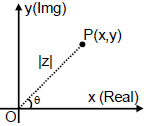Length OP is called modulus of the complex number denoted by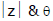is called the argument or amplitude.
eg.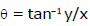(angle made by OP with positive x-axis)

Remark :
(i)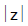is always non negative . Unlike real numbers=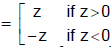is not correct

(ii) Argument of a complex number is a many valued function .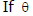is the argument of a complex number then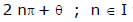will also be the argument of that complex number. Any two arguments of a complex number differ by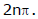(iii) The unique value of θ such that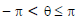is called the principal value of the argument.

(iv) Unless otherwise stated, amp z implies principal value of the argument.

(v) By specifying the modulus & argument a complex number is defined completely. For the complex number 0 + 0 i the argument is not defined and this is the only complex number which is given by its modulus.

(vi) There exists a one-one correspondence between the points of the plane and the members of the set of complex numbers.

(b) Trigonometric / Polar Representation :

z = r (cos θ+ i sin θ)   where |z| = r   ;   arg  z  = θ  ;   z- =  r (cos θ - i sin θ)

Remark : cos θ + i sin θ  is also written as  CiS θ  .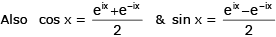are known as Euler's identities.

Ex.14 Express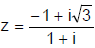in polar form and then find the modulus and argument of z. Hence deduce the value of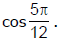Sol.

Let –1 + i √3 = r(cosθ + i sinθ). Equating real and imaginary parts, r cosθ = -1, r sinθ= √3 .

Now r2 = 1 + 3 = 4, r = 2, cosθ = -1/2, sinθ √3/2, or θ = 2π/3 between –π and π.

Consequently, –1 + i √3 =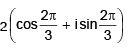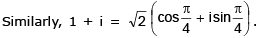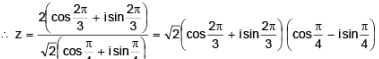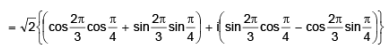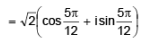It is the polar form of z. Obviously, |z| = √2 and arg z = 5/12 (principal value).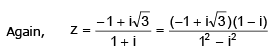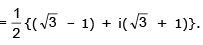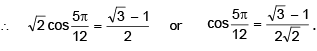(c) Exponential Representation :

z = reiθ  ;  |z| = r   ;   arg z  =  θ ;   z- = re- iθ

(d) Vectorial Representation :Every complex number can be considered as if it is the position vector of that point. If the point P represents the complex number z then,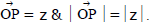Ex.15 If (1 – i) is a root of the equation , z3 - 2 (2 - i) z2 + (4 - 5 i) z - 1 + 3 i = 0 then find the other two roots.

Sol.

z1 + z2 + z3 = 2 (2 – i) ⇒ z+ z= 3 - i ( z1 = 1 - i) .......(1)

again z1 z2 z3 = 1 – 3 i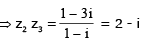......(2)

From (1) & (2) z2 = 1 & z3 = 2 – i

Ex.16 Prove that if the ratio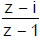is purely imaginary then the point z lies on the circle whose centre is at the point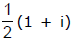and radius is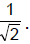Sol. Let z = x + iy.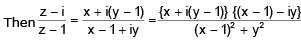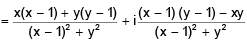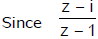is purely imaginary, x(x – 1) + y(y – 1) = 0 or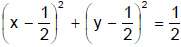It is a circle with radius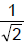and centre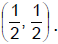Therefore, the point z lies on a circle and the centre is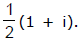Ex.17 A function f is defined on the complex number by f (z) = (a + bi)z, where 'a' and 'b' are positive numbers. This function has the property that the image of each point in the complex plane is equidistant from that point and the origin. Given that | a + bi | = 8 and that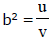where u and v are co-primes. Find the value of (u + v).

Sol. Given | (a + bi)z – z | = | (a + bi) z | ⇒ | z(a – 1) + biz | = | az + bzi |

= | z | | (a – 1) + bi | = | z | | a + bi |

⇒(a – 1)2 + b2 = a2 + b2  ∴ a = 1/2

since | a + bi | = 8

= a2 + b2 = 64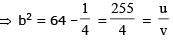∴  u = 255 & v = 4   ⇒  u + v = 259

Ex.18 Show that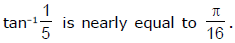Sol.
We have (5 + i) = 26 (cosθ + isinθ), where tanq = 1/5 and therefore (5 + i)4 = 676(cos4θ + isin 4θ).
But (5 + i)4 = (24 + 10i) = 476 + 480i ; hence we have

cos 4θ = 476/676, sin 4θ = 480/676, and tan 4θ = 1, nearly.

∴ 4θ = π/4 approximately.

Ex.19 If a & b are complex numbers then find the complex numbers z1 & z2 so that the points z1 , z2 and a, b be the corners of the diagonals of a square .

Sol.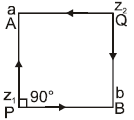a - z1 = (b - z1) e i p/2 = i (b - z1)

a - i b = z1 (1 - i)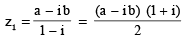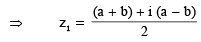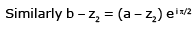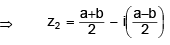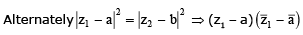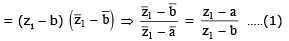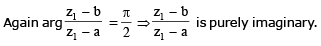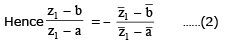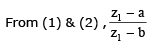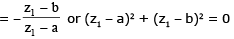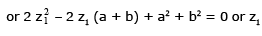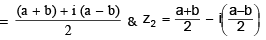Ex.20 Find the square root of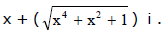Sol.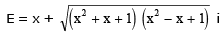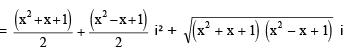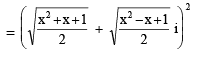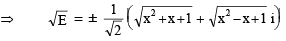Ex.21 On the Argand plane point 'A' denotes a complex number z1 . A triangle OBQ is made directly similar to the triangle OAM, where OM = 1 as shown in the figure . If the point B denotes the complex number z2, then find the complex number corresponding to the point 'Q' in terms of z1 & z2 .
Sol .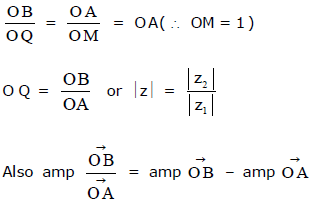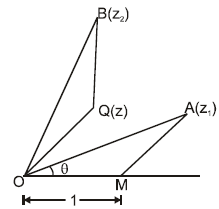=  ∠ BOM  - ∠ AOM =  ∠ BOM  - ∠ BOQ =  ∠ QOM =  amp  of  z ( ∠ AOM = ∠ BOQ = θ) Hence complex number corresponding to the point  Q = Z2/Z1

Ex.22 Compute the product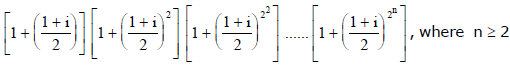Sol. Assume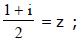multiply numerator and denominator by (1 - z) which simplifies to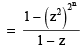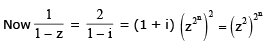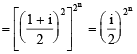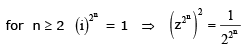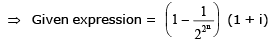Ex.23 Find the set of points on the complex plane such that z2 + z + 1 is real and positive (where z = x + i y) .

Sol. x2 - y2 + 2xy i + x + i y + 1  is real and positive ⇒ (x2 - y2 + x + 1) +  y (2x + 1) i  is real and positive ⇒ y (2x + 1) = 0  and  x2 - y2 + x + 1 > 0  if  y = 0  then  x2 + x + 1 is always positive

complete  x - axis    if  x = -1/2 then 3/4 - y2 >0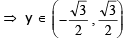The document Representation of a Complex Number | Mathematics (Maths) Class 11 - Commerce is a part of the Commerce Course Mathematics (Maths) Class 11.
All you need of Commerce at this link: Commerce

## Mathematics (Maths) Class 11

156 videos|176 docs|132 tests

## Mathematics (Maths) Class 11

156 videos|176 docs|132 tests

Track your progress, build streaks, highlight & save important lessons and more!(Scan QR code)

,

,

,

,

,

,

,

,

,

,

,

,

,

,

,

,

,

,

,

,

,

;